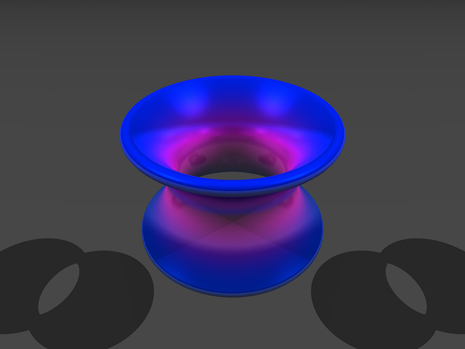## Chapter 6 Review Excercises

True or False? Justify your answer with a proof or a counterexample.

1. The amount of work to pump the water out of a half-full cylinder is half the amount of work to pump the water out of the full cylinder.

2. If the force is constant, the amount of work to move an object from $x=a$ to $x=b$ is $F(b-a).$

3. The disk method can be used in any situation in which the washer method is successful at finding the volume of a solid of revolution.

4. If the half-life of $\text{seaborgium-}266$ is 360 ms, then $k=(\text{ln}(2))\text{/}360.$

For the following exercises, use the requested method to determine the volume of the solid.

5. The volume that has a base of the ellipse ${x}^{2}\text{/}4+{y}^{2}\text{/}9=1$ and cross-sections of an equilateral triangle perpendicular to the $y\text{-axis}\text{.}$ Use the method of slicing.

6. $y={x}^{2}-x,$ from $x=1\text{ to }x=4,$ rotated around the$y$-axis using the washer method

7. $x={y}^{2}$ and $x=3y$ rotated around the $y$-axis using the washer method

8. $x=2{y}^{2}-{y}^{3},x=0,\text{ and }y=0$ rotated around the $x$-axis using cylindrical shells

For the following exercises, find

1. the area of the region,
2. the volume of the solid when rotated around the $x$-axis, and
3. the volume of the solid when rotated around the $y$-axis. Use whichever method seems most appropriate to you.

9. $y={x}^{3},x=0,y=0,\text{ and }x=2$

10. $y={x}^{2}-x\text{ and }x=0$

11. [T]$y=\text{ln}(x)+2\text{ and }y=x$

12. $y={x}^{2}$ and $y=\sqrt{x}$

13. $y=5+x,$$y={x}^{2},$$x=0,$ and $x=1$

14. Below ${x}^{2}+{y}^{2}=1$ and above $y=1-x$

15. Find the mass of $\rho ={e}^{\text{−}x}$ on a disk centered at the origin with radius 4.

16. Find the center of mass for $\rho ={ \tan }^{2}x$ on $x\in (-\frac{\pi }{4},\frac{\pi }{4}).$

17. Find the mass and the center of mass of $\rho =1$ on the region bounded by $y={x}^{5}$ and $y=\sqrt{x}.$

For the following exercises, find the requested arc lengths.

18. The length of $x$ for $y=\text{cosh}(x)$ from $x=0\text{ to }x=2.$

19. The length of $y$ for $x=3-\sqrt{y}$ from $y=0$ to $y=4$

For the following exercises, find the surface area and volume when the given curves are revolved around the specified axis.

20. The shape created by revolving the region between $y=4+x,$ $y=3-x,$ $x=0,$ and $x=2$ rotated around the $y$-axis.

21. The loudspeaker created by revolving $y=1\text{/}x$ from $x=1$ to $x=4$ around the $x$-axis.

For the following exercises, consider the Karun-3 dam in Iran. Its shape can be approximated as an isosceles triangle with height 205 m and width 388 m. Assume the current depth of the water is 180 m. The density of water is 1000 kg/m ${}^{3}.$

22. Find the total force on the wall of the dam.

23. You are a crime scene investigator attempting to determine the time of death of a victim. It is noon and $45\text{°}\text{F}$ outside and the temperature of the body is $78\text{°}\text{F}.$ You know the cooling constant is $k=0.00824\text{°}\text{F/min}\text{.}$ When did the victim die, assuming that a human’s temperature is $98\text{°}\text{F}$
?

For the following exercise, consider the stock market crash in 1929 in the United States. The table lists the Dow Jones industrial average per year leading up to the crash.

Source: http://stockcharts.com/freecharts/historical/djia19201940.html
Years after 1920 Value (\$)
1 63.90
3 100
5 110
7 160
9 381.17

24. [T] The best-fit exponential curve to these data is given by $y=40.71+{1.224}^{x}.$ Why do you think the gains of the market were unsustainable? Use first and second derivatives to help justify your answer. What would this model predict the Dow Jones industrial average to be in 2014
?

For the following exercises, consider the catenoid, the only solid of revolution that has a minimal surface, or zero mean curvature. A catenoid in nature can be found when stretching soap between two rings.

25. Find the volume of the catenoid $y=\text{cosh}(x)$ from $x=-1\text{ to }x=1$ that is created by rotating this curve around the $x\text{-axis},$ as shown here.26. Find surface area of the catenoid $y=\text{cosh}(x)$ from $x=-1$ to $x=1$ that is created by rotating this curve around the $x\text{-axis.}$